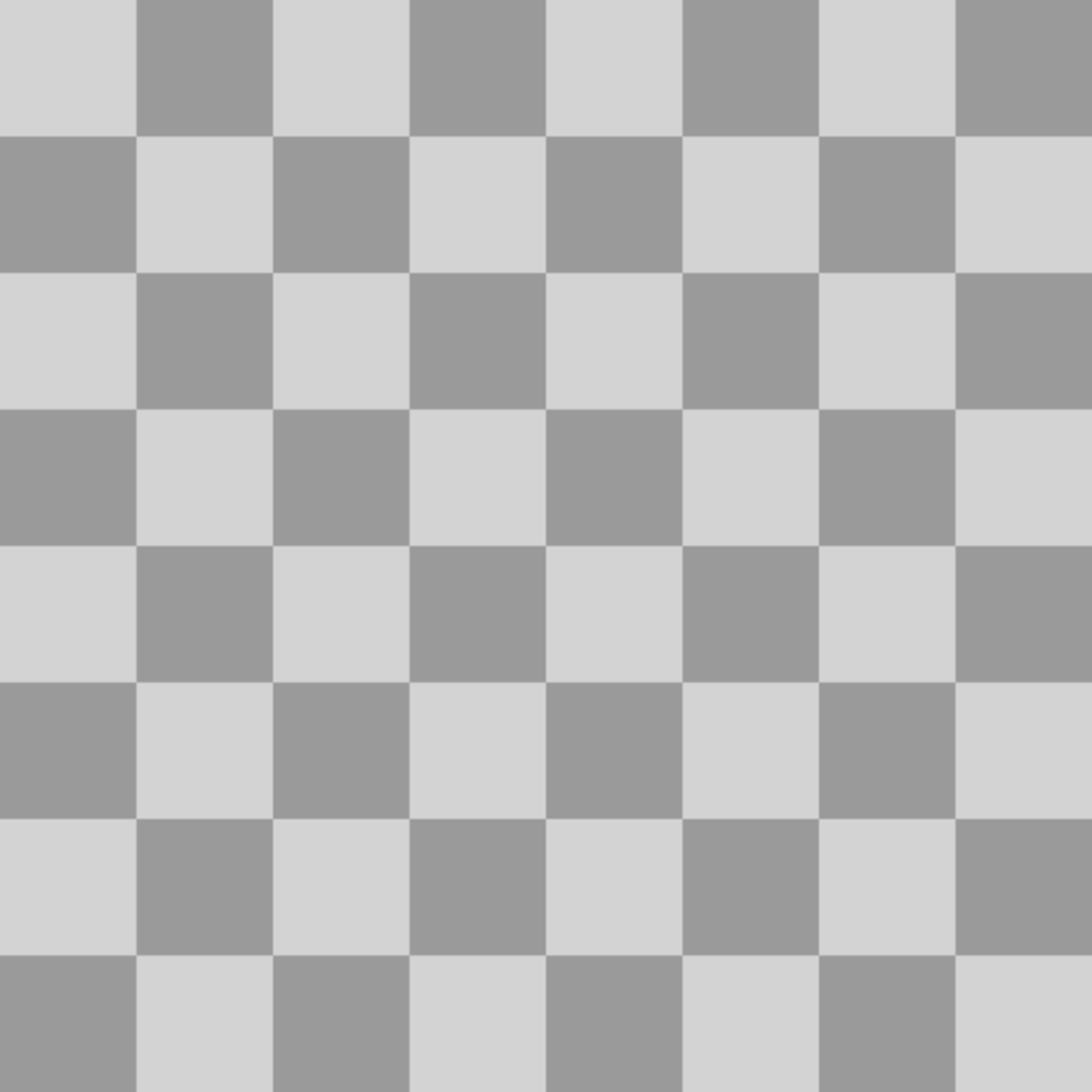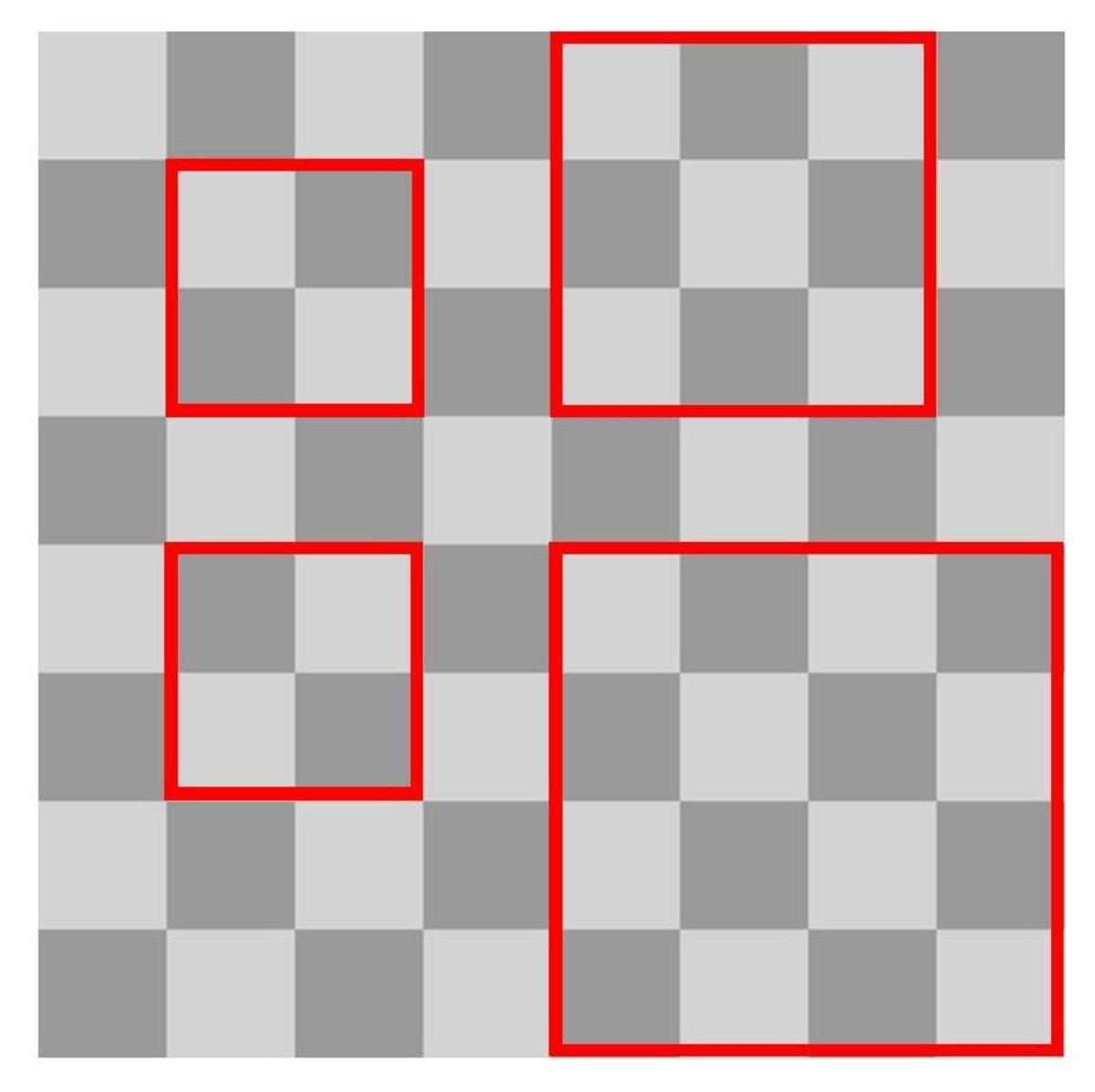# How Many Squares Are on a Chessboard? A Maths Problem

• Author:
• Updated date:

I am a former maths teacher and owner of DoingMaths. I love writing about maths, its applications, and fun mathematical facts.The total number of squares on a chessboard can be calculated using a formula.

## How Many Squares Are There on a Normal Chessboard?

So how many squares are there on a normal chessboard? 64? Well, of course that is the correct answer if you are only looking at the small squares inhabited by the pieces during a game of chess or draughts/checkers. But what about the larger squares formed by grouping these small squares together? Look at the diagram below to see more.Assorted squares on a standard chessboard

## Different Sized Squares on a Chessboard

You can see from this diagram that there are many different squares of various sizes. To go with the single squares there are also squares of 2x2, 3x3, 4x4 and so on up until you reach 8x8 (the board itself is a square too).

Let's have a look at how we can count these squares, and we'll also work out a formula to be able to find the number of squares on a square chessboard of any size.

## The Number of 1x1 Squares

We have already noted that there are 64 single squares on the chessboard. We can double-check this with a bit of quick arithmetic. There are 8 rows and each row contains 8 squares, hence the total number of individual squares is 8 x 8 = 64.

Counting the total number of larger squares is a little bit more complicated, but a quick diagram will make it a lot easier.

## How Many 2x2 Squares?

Look at the diagram above. There are three 2x2 squares marked on it. If we define the position of each 2x2 square by its top-left corner (denoted by a cross on the diagram), then you can see that to remain on the chessboard, this crossed square must remain within the shaded blue area. You can also see that each different position of the crossed square will lead to a different 2x2 square.

The shaded area is one square smaller than the chessboard in both directions (7 squares) hence there are 7 x 7 = 49 different 2x2 squares on the chessboard.

## How Many 3x3 Squares?

The diagram above contains three 3x3 squares, and we can calculate the total number of 3x3 squares in a very similar way to the 2x2 squares. Again, if we look at the top-left corner of each 3x3 square (denoted by a cross) we can see that the cross must stay within the blue shaded area in order for its 3x3 square to remain completely on the board. If the cross was outside of this area, its square would overhang the edges of the chessboard.

The shaded area is now 6 columns wide by 6 rows tall, hence there are 6 x 6 = 36 places where the top-left cross can be positioned and 36 possible 3x3 squares.A chessboard with a 7x7 square
Scroll to Continue

## What About the Rest of the Squares?

To calculate the number of larger squares, we proceed in the same way. Each time the squares we are counting get bigger, i.e., 1x1, 2x2, 3x3, etc., the shaded area that the top left part sits in becomes one square smaller in each direction until we reach the 7x7 square seen in the picture above. There are now only four positions that 7x7 squares can sit in, again denoted by the top-left crossed square sitting within the shaded blue area.

## The Total Number of Squares on the Chessboard

Using what we have worked out so far we can now calculate the total number of squares on the chessboard:

• Number of 1x1 squares = 8 x 8 = 64
• Number of 2x2 squares = 7 x 7 = 49
• Number of 3x3 squares = 6 x 6 = 36
• Number of 4x4 squares = 5 x 5 = 25
• Number of 5x5 squares = 4 x 4 = 16
• Number of 6x6 squares = 3 x 3 = 9
• Number of 7x7 squares = 2 x 2 = 4
• Number of 8x8 squares = 1 x 1 = 1

The total number of squares = 64 + 49 +36 + 25 + 16 + 9 + 4 + 1 = 204

## What About Larger Chessboards?

We can take the reasoning that we have used so far and expand upon it to create a formula for working out the number of squares possible on any size of square chessboard.

If we let n represent the length of each side of the chessboard in squares then it follows that there are n x n = n2 individual squares on the board, just like there are 8 x 8 = 64 individual squares on a normal chessboard.

For 2x2 squares, we have seen that the top left corner of these must fit into a square that is one smaller than the original board, hence there are (n - 1)2 2x2 squares in total.

Each time we add one to the side length of the squares, the blue-shaded area that their corners fit into shrinks by one in each direction. Therefore there are:

• (n - 2)2 3x3 squares
• (n - 3)2 4x4 squares

You can continue this calculation until you get to the final, large square that is the same size as the whole chessboard.

In general, you can quite easily see that for an n x n chessboard the number of m x m squares will always be (n - m + 1).

So for an n x n chessboard, the total number of squares of any size will equal n2 + (n - 1)2 + (n - 2)2 + . . . + 22 + 12or, in other words, the sum of all the square numbers from n2 down to 12.

Example: The total number of squares on a 10 x 10 chessboard would be 100 + 81 + 64 + 49 + 36 + 25 + 16 + 9 + 4 + 1 = 385.

## Something to Think About

What if you had a rectangular chessboard with sides of different lengths? How can you expand our reasoning so far to come up with a way of calculating the total number of squares on an n x m chessboard?